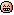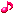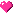other initiatives < fweight fsize span bbox_x = "276" bbox_y = "1051" bbox_w = "272" bbox_h = "24" = "16" = "3" red = "81" green = "39" blue = "2" alpha = "255" > Bon plan?

< fweight fsize span bbox_x = "276" bbox_y = "1103" bbox_w = "352" bbox_h = "24" = "16" = "3" red = "81" green = "39" blue = "2" alpha = "255" > still will have decided not come to toho-enhot < fweight fsize = span bbox_x = "276" bbox_y = "1153" bbox_w = "528" bbox_h = "49" "16" = "3" red = "81" green = "39" blue = "2" alpha = "255" >, took a cooler room, laid-back, want to spend enjoyable holidays of< fweight fsize span bbox_x = "276" bbox_y = "1203" bbox_w = "256" bbox_h = "24" = "16" = "3" red = "81" green = "39" blue = "2" alpha = "255" > please come and do their< fweight fsize span bbox_x = "276" bbox_y = "1253" bbox_w = "512" bbox_h = "24" = "16" = "3" red = "81" green = "39" blue = "2" alpha = "255" > father and I along with my mother and child was cut, just jump into the riverchannels < fweight fsize span bbox_x = "276" bbox_y = "1328" bbox_w = "320" bbox_h = "24" = "16" = "3" red = "81" green = "39" blue = "2" alpha = "255" > I cannot see the child's growth or even